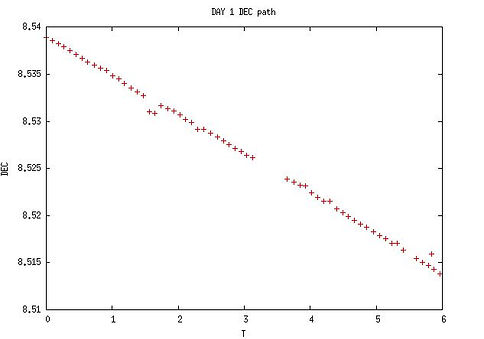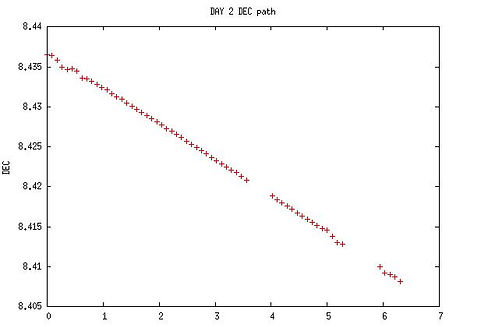top of page

## Calculation of the distance to a comet using the technique of daytime parallax

###### Introduction

One of the calculations that are available to the amateur astronomer is the use of daytime parallax to calculate the distance of a comet or asteroid. The diurnal parallax takes advantage of the fact that the coordinates of a star vary depending on which point of the earth's surface the measurements are made. The observer with his telescope is moving throughout the night and this difference is appreciable for stars not far away.

In this case, for this project I have chosen a comet with a trajectory very close to Earth, approximately 0.36UA on the date of measurements on 5/20/2016.

###### Methodology

The methodology is inspired by the book "Astronomical Discoveries you can make, too! Replicating the Work of the Great Observers" by Robert K. Buchheim, Project 25, although some variations have been introduced.

The idea is to measure the RA and DEC coordinates of the comet during 2 days (in the book it is explained with 3 days, but at least for a near comet with 2 days it works perfectly) in small intervals.

Parallax is measured as the angular difference between geocentric and topocentric RA. The RAs that we can measure with the CCD are the topocentric ones, since we measure from the surface of the Earth. However, it is possible to calculate the geocentric coordinates for all the photos by means of a trick that is based on the geocentric and topocentric coordinates RA and DEC coinciding in the transit through the meridian:

1. The exact hour, RA and DEC values ​​of the transit by the meridian of the star on both nights are calculated. Thus we obtain at least 2 values ​​of geocentric ra and dec.

2. The speed of change of geocentric RA and DEC is calculated by dividing the difference of the values ​​obtained from the time elapsed between the transits. For the calculation of the transit it is taken into account that this occurs when the RA match with the sidereal time, so the way to do the calculation is to have the intersection of the line that best approximates the measured values ​​of RA with the straight line of the sidereal time. For the moment obtained in which the intersection occurs, the calculation of the transition for DEC is made.

3. It is estimated that the geocentric RA and DEC of for example the first night is the value in transit plus the rate of change for time.

Once we have RA and DEC both topocentric (the measured values) and geocentric (the calculated values) the difference is made and we obtain the parallax. Since the parallax responds to a theoretical formula that depends on the distance from the Earth to the star, iterations with different values ​​of the distance are made until obtaining the best approximation (minimum quadratic error).

This procedure may seem a little complicated, but it is better understood with the graphs and explanations shown below.

###### Tools

Capture of the photos: C11 telescope with focal reducer f6.3 on mount EQ8, guided Off Axis with Lodestar X2, camera ST8xME, without filter. Software used: Maxim DL.

Automation: the photos were taken continuously for 2 nights with captures of 5 minutes each. The automation of the capture process was carried out with ACP DC3 software, which also resolves each image astrometrically.

Astrometric calculations: once the photos are referenced astrometrically during the capture process, it is necessary to extract for each photo the time, straight ascension and decline of the comet / asteroid. The process is a little tedious, and consists of opening each photo with Maxim DL, and use the following windows:

• "FITS header" to extract with precision seconds the start time of the photo

• "Toogle Information" (selected "Mode Astrometric") be placed manually on the comet / asteroid and note the RA and DEC of the centroid.

These data are recorded in a spreadsheet with the columns: photo number, date, time, RA and DEC. It is important to note that for the hour, RA and DEC, the data must be recorded with precision of seconds.

Parallax calculations: for the calculation of the parallax and consequently the distance, the spreadsheet can be used directly but in my case I preferred to export the astrometric data to 2 files with CSV format, one for each day, and make a simple program in C that makes the calculations and the graphic representation using GNUPLOT tool was used under Cygwin.

###### Formulas used

The following formulas are used:

• Geocentric RA rate of change:

• v_ra = (ra_transit_day2 - ra_transit_day1)  / (hour_transit_day2 - hour_transit_day1)

• Geocentric DEC rate of change:

• v_dec = (dec_transit_day2 - dec_transit_day1)  / (hour_transit_day2 - hour_transit_day1)

• RA geocentric: (during day 1)

• ra_geocentric = ra_transit_day1 + t * v_ra

• DEC geocentric: (during day1)​

• dec_geocentric = dec_transit_day1 + t * v_dec​

• Parallax angle:​ (during day 1)

• Angel in hours = ​(ra_topocentric - ra_geocentric) * cos (dec_geocentric)

• Theoretical parallax angle:​

• Angel in radians = (radius_month * cos (latitude) / distance _astro) * sin ( 15.04 * t)

###### Comet 252P/Linear

The choice of Comet 252P / Linear is based on its proximity to the Earth, about 0.36UA, and its visibility at this time during an important part of the night with magnitude around 9. In the photo shown below, the comet appears very dim in the center of the image.###### RA and DEC data obtained

On day 1 (5/20/2016) about 60 photos of 5 minutes duration were taken, without filter. For each photo, once resolved astrometrically, the position in RA and DEC of the centroid of the head of the comet was obtained with Maxim DL, and the following graphs show the results obtained:In both graphs the abscissas are the hours relative to the time of the first photo. A hole is observed due to the telescope turning to the meridian, which, being with an equatorial mount, I have configured not to take pictures a few minutes before and after to avoid colliding the camera with the pier.

Precisely in those gaps of measurements is where the transit of the comet, but it is not a problem since the technique of calculation of traffic based on using the line that best fits the measures. In fact, some errors are observed in the measures that will not have effect thanks to this technique.In both graphs, the line that best fits the measurements (in red) is represented, as well as:

• In the case of the RA graph, the straight line is also represented as the local sidereal time values. When both lines intersect, the right ascension match with the local sidereal time, and this happens precisely in the transit through the meridian. The value obtained is RA = 16,949186 (16:56:57).

• In the case of the DEC graph, it is calculated the value of the line at the time previously calculated for the meridian transition. The value obtained is DEC =8.525301 (8:31:31).

The calculations are repeated for day 2:The data obtained for day 2 are:

• Transit by the meridian RA = 16.931770 (16:55:54) = RA geocentric at the time of transit

• Transit by the meridian DEC = 8.420037 (8:25:12) = DEC geocentric at the time of transit

###### Results

From the data of day1 and day2 the change rates of the RA and geocentric DEC are calculated by dividing the difference of RA and DEC respectively between the time elapsed between the two transits, obtaining:

• Rate of change of the geocentric RA = -0.000726 hour / hour

• Rate of change of the geocentric DEC = -0.004389 degrees / hour

With these data we can calculate the RA and DEC geocentric throughout the first day. The parallax angle is the difference of the geocentric RA (calculated from the geocentric RA at the time of transit through the meridian plus the product of time by the calculated rate of change) minus the topocentric RA (measured with the CCD photos) and this difference is multiplied by the cosine of the geocentric DEC.

In the following graph the data obtained from parallax are shown by points:The abscissa represents the hours with respect to the transit time. The ordinates represent the parallax in arcs of seconds.

The points correspond to a sine wave and the way to calculate the distance of the comet from the Earth is to deduce the amplitude of the sine curve that makes the calculated curve correspond to the theoretical curve. In the previous section of formulas it is indicated in which specific form the amplitude depends on the distance to the comet.

An iteration has been made by varying the distance in each step by 250,000km and it has been obtained that the best value (minimum quadratic error) between the measured data and the theoretical curve is found for a distance of 54,250,000 km, equivalent to 0.362 UA.

The graph shows three theoretical curves: for 44,000,000 km, 64,000,000 km and 54,250,000 km.

Since the adjustment is maximum, we deduct as obtained data 54.250.000 km

bottom of page